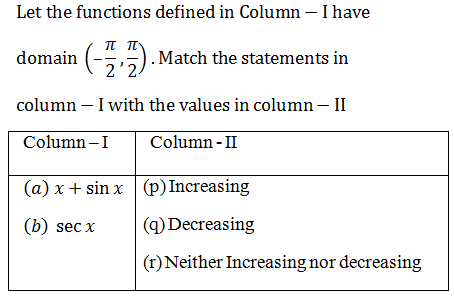In the section each question has some statements (A, B,C,D,…) given in Column – I and some statements (p,q,r,s,t,…) in column – II. Any given statement is column – I can have correct matching with ONE OR MORE statement(s) in column – II for example, if for a given question, statement B matches with the statements given in q and r, then for that particular question against statement B, darken the bubbles corresponding to q and r in the ORS. i.e., answer r will be q and r1) A → p; B → r

2) A → r; B → q

3) A → p; B → q

4) A → q; B → r

Solution: (1) A → p; B → r

A → p; B → r

(A) f (x) = x + sin x

f’ (x) = 1 + cos x > 0, for all x ∈ (-π / 2, π / 2)

f (x) is increasing.

(B) Let g (x) = sec x

g’ (x) = sec x tan x, which is positive if 0 < x < π / 2 and negative if -π / 2 < x < 0

g (x) is neither increasing nor decreasing.(0)(0)